#Quality Digest

Featured Product
This Week in Quality Digest Live
Statistics Features
Donald J. Wheeler
What does this ratio tell us?
Harish Jose
Any statistical statement we make should reflect our lack of knowledge
Donald J. Wheeler
How to avoid some pitfalls
Kari Miller
CAPA systems require continuous management, effectiveness checks, and support
Donald J. Wheeler
What happens when the measurement increment gets too large?
Statistics News
How to use Minitab statistical functions to improve business processes
New capability delivers deeper productivity insights to help manufacturers meet labor challenges
Day and a half workshop to learn, retain, and transfer GD&T knowledge across an organization
Elsmar Cove is a leading forum for quality and standards compliance
InfinityQS’ quality solutions have helped cold food and beverage manufacturers around the world optimize quality and safety
User friendly graphical user interface makes the R-based statistical engine easily accessible to anyone
Collect measurements, visual defect information, simple Go/No-Go situations from any online device
Good quality is adding an average of 11 percent to organizations’ revenue growth
Ability to subscribe with single-user minimum, floating license, and no long-term commitmentBio

Statistics

## Problems With the AIAG Gauge R&R Study

### And how to fix them so as to avoid the nonsense

Published: Tuesday, July 6, 2021 - 12:03

Measurement error is ubiquitous. As a result, over the past 250 years, different areas of science and engineering have come up with many different ways to deal with the problem of measurement error. One approach to the problem of measurement error was developed during the 1960s within General Motors. Over the years it was modified and revised until, in 1989, it was turned over to the Automotive Industry Action Group (AIAG). Since that time the AIAG Gauge R&R Study has been promoted throughout many different industries. Unfortunately, the original procedure contained some fundamental problems that have not been corrected over the years. This column will address these historic problems and suggest solutions.

### The AIAG Gauge R&R Study

The AIAG Gauge R&R Study starts out with a sound strategy for collecting data. A simple fully crossed experiment is performed where two or more operators measure each of three to 10 parts two or three times apiece. To have an example to use we shall use the data shown in figure 1 where three operators measure each of five parts two times apiece.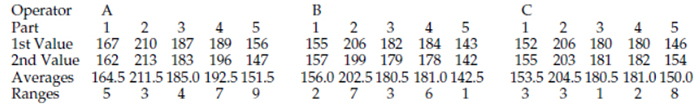Figure 1: The gasket thickness data

The average and range are shown for each of the 15 pairs of measurements in figure 1. Since each of these pairs represents the same operator measuring the same part, the ranges characterize the basic component of measurement error. This component has many names. Among these are test-retest error, repeatability, and equipment variation. This component may be estimated by dividing the average range by the appropriate bias correction factor d2:Next, under the assumption that the operators are different, an estimate of the reproducibility is obtained by computing an average value for each operator and finding the range of these averages. Here the operator averages are 181.0, 172.5, and 173.9 and the range of these three averages is Ro = 8.5 mils. This range value is divided by a bias correction factor for the range of three values, d2* = 1.906, and then, with o = number of operators, p = number of parts, and n = number of repeated measurements, the reproducibility is estimated to be: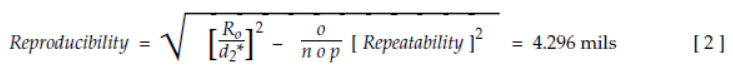Once we have an estimate of both the repeatability and the reproducibility we can combine them to estimate the combined R&R component as:Next the product variation is estimated by computing averages for each of the p parts and using the range, Rp. Here the five part averages are 158.0, 206.167, 182.0, 184.833, and 148.0. The range for these part averages is Rp = 58.167. The bias correction factor used is d2* = 2.477, which is for one range of five values. The product variation is estimated to be:Finally, the total variation for the set of product measurements is estimated by combining the repeatability, the reproducibility, and the product variation to get:Up to this point everything is okay. While the estimators defined here are not the only formulas that could have been used, and while they are not always unbiased estimators, they do provide reasonable estimates for the quantities described. (While the AIAG Gauge R&R computational worksheet simplifies the formulas by defining several “K-factors” the AIAG formulas are algebraically equivalent to formulas  through .)

The train wreck begins when the AIAG Gauge R&R Study tries to use the estimates from formulas  through  to characterize relative utility. In the current version the first four quantities are all expressed as a percentage of the total variation from formula .

### The “percentages” of the total variation

The repeatability  is divided by the total variation  and multiplied by 100 to be expressed as a percentage. This ratio is said to represent the per­centage of the total variation that is consumed by repeatability. Here we find: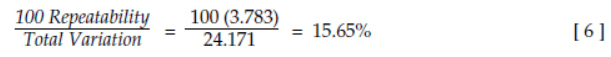Next the reproducibility  is divided by the total variation  and multiplied by 100. This ratio is said to represent the percentage of the total variation that is consumed by reproducibility. Here we find:The combined repeatability and reproducibility  is divided by the total variation  and multiplied by 100. This ratio is said to represent the per­centage of the total variation that is consumed by the combined repeatability and reproducibility. Here we find:Finally, the product variation  is divided by the total variation  and multiplied by 100. This ratio is said to represent the per­centage of the total variation that is consumed by the product variation. Here we find:Following these ratios, the current version of the AIAG manual has a simple statement to the effect the “The sum of the percent consumed by each factor will not equal 100%.” This statement has no explanation attached. There is no guidance offered on how to proceed now that common sense and every rule in arithmetic have been violated. Just a simple statement that these numbers do not mean what they were just interpreted to mean, and the user is left to his or her own devices. Unfortunately, unlike the Red Queen in Won­derland, when it comes to arithmetic we do not get to say that things mean whatever we want them to mean.

### Why these “percentages” do not add up

The repeatability “percentage” of formula  and the reproducibility “percentage” of formula  do not add up to the combined R&R “percentage” of formula  because they are not based on proportions. Likewise, the combined R&R “percentage” of formula  and the product variation “percentage” of formula  do not add up to 100 percent because they are not based on proportions. Instead of being proportions, it turns out that the ratios computed above are all trigonometric functions. Figure 2 shows how the five estimates of variation are related.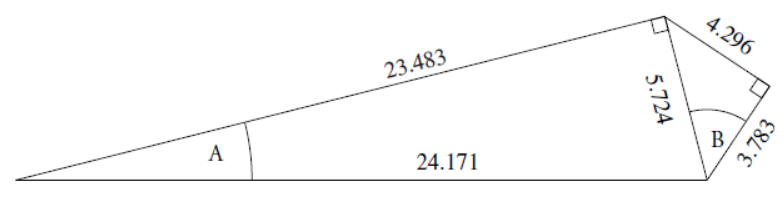Figure 2: The five estimates of variation

Thus, based on figure 2, and recalling some of our high school trigonometry, we see that the ratio of the repeatability to the total variation is: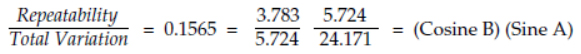The ratio of the reproducibility to the total variation is: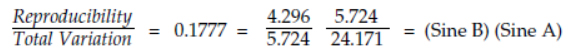The ratio of the combined repeatability and reproducibility to the total variation is:And the ratio of the product variation to the total variation is: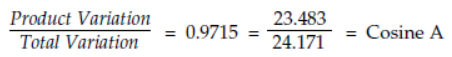In this form we can begin to see why these quantities do not add up. While they were dressed up to look like percentages, and while they were interpreted as percentages, they are, and always have been, nothing more than trigonometric functions. And trigonometric func­tions do not satisfy the conditions required for a set of ratios to be interpreted as proportions or percentages.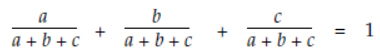A set of ratios will be proportions only when the denominator is the sum of the numerators. This additivity of the numerators is the essence of proportions. So what is additive in a gauge R&R study? Look at the structure of formula . It is the variances that are additive:Since these variances are additive, we know from the Pythagorean theorem that the standard deviations cannot be additive. However, the ratios computed by the AIAG Gauge R&R Study implicitly assume that the standard deviations are additive. This implicit assumption of additivity is a violation of the Pythagorean theorem, and is what makes it impossible to make sense of the ratios in the AIAG Gauge R&R Study. This is why the “percentages” of ratios  through  do not add up, and this is why engineers have told me they never could figure out exactly what the final numbers in a Gauge R&R Study repre­sented. The ratios sound like nonsense simply because it is nonsensical to interpret trigonometric functions as proportions.

### So what are the actual proportions?

Formula  above suggests the solution to the problem of how to characterize the contribution of the various components to the total variation in the product measurements. For example, the repeatability contribution to the total variation could be estimated by: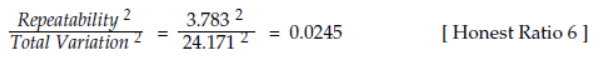which is 2.45 percent rather than the 15.65 percent erroneously found earlier. The reproducibility contribution to the total variation could be estimated by:which is 3.16 percent rather than the 17.77 percent erroneously found earlier. The combined repeatability and reproducibility contribution to the total variation could be estimated by: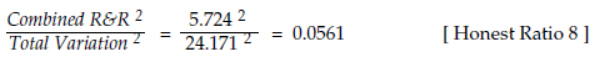which is 5.61 percent rather than the 23.68 percent erroneously found earlier. We also should note that this 5.61 percent is the sum of the 2.45 percent and the 3.16 percent, which is what we expect when computing proportions. The product variation contribution to the total variation could be estimated by: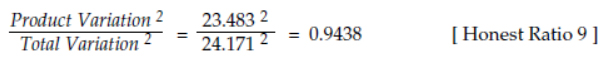which is 94.38 percent rather than the 97.15 percent erroneously found earlier. When this value is added to the combined R&R percentage we effectively get 100 percent. Now we have correctly accounted for the various components of the variation in the product measurements.

Honest Ratio  is the traditional measure of relative utility introduced by Sir Ronald Fisher in 1921. In this context it is commonly known as the intraclass correlation coefficient (ICC). Here the value of 0.9438 tells us that over 94 percent of the variation in the product measurements is attributable to the variation in the product stream, and less than 6 percent of the variation in the product measurements is attributable to variation in the measurement system (combined R&R). Thus, this one number summarizes the essential information contained in all four of the honest ratios above and provides a characterization of the relative utility of the measurement system for measuring a particular product.

### So what should you do?

Since any attempt to use ratios  through  from the AIAG Gauge R&R Study is a violation of the Pythagorean theorem, you will need to convert the ratios into the correct quantities shown as honest ratios [Honest Ratio 6] through [Honest Ratio 9] before attempting to interpret or use them in any manner. No exceptions. No loopholes. No excuses.

### The “percentages” of the specified tolerance

Ratios  through  were added to the GM Gage [sic] R&R Study in its 1984 revision. Prior to 1984 there were only three ratios. These compared the repeatability , the reproducibility , and the combined R&R  to the specified tolerance.

Assume that the specifications for the gaskets of figure 1 are 145 mils to 225 mils. How much of this specification range is “lost” due to measurement error? In an attempt to answer this question the GM Gage R&R Study multiplied the repeatability by 5.15 (presumably to account for 99% of the measurement error), divided by the specified tolerance, and then multiplied by 100 to express the result as a percentage. In an attempt to account for more than 99% of the measurement error, the AIAG Gauge R&R Study eventually changed the initial multiplier from 5.15 to 6.00. Thus, the following ratio is said to represent that amount of the specified tolerance that is consumed by repeatability: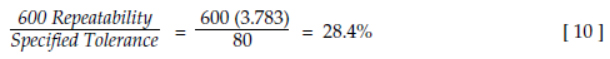In a similar manner, reproducibility is said to consume the following amount of the specified tolerance: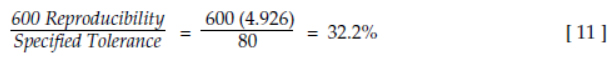However, the combined repeatability and reproducibility is said to consume the following amount of the specified tolerance: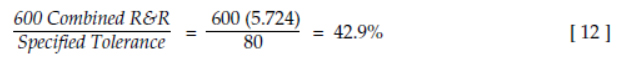Once again, these three ratios do not add up. Moreover, since reproducibility cannot occur without repeatability, formula  is merely an academic exercise without any practical use.

Formulas  and  are versions of what are popularly known as precision to tolerance (P/T) ratios. The values of 28.43% and 42.9% found here would erroneously be classed by the AIAG study as being respectively “marginal” and “unacceptable.” However, as we learned last month in “More About the Precision to Tolerance Ratio” (Quality Digest, June 1, 2021), we cannot use these P/T ratios to condemn any measurement process. While P/T ratios that are less than 10% are good, the converse is not true. Here, with an interclass correlation of 0.94, a P/T ratio of 28% corresponds to a potential process yield of 90% good product produced and shipped. With the larger P/T ratio of 43% we would estimate a potential process yield of 88% good product produced and shipped.

### The “number of distinct categories”

Finally, the AIAG Gauge R&R Study computes a quantity it calls the “number of distinct categories” (ndc) using the formula:The AIAG manual suggests rounding this value off to an integer, and that values of 5 or greater are “good.” (Simple algebra will show that this value is inconsistent with the guidelines given below, but inconsistencies of this sort are found throughout the AIAG manual.)

The citation given in the AIAG manual for formula  is the First Edition of Evaluating the Measurement Process by this author and Richard Lyday. In that text we defined a quantity which we called the classification ratio, and the formula above does provide an estimate of this classification ratio. However, nowhere in that text did we ever suggest that this ratio would define the number of distinct categories.

Unfortunately, in practice there is no simple interpretation for the classification ratio. While the classification ratio does approximate the relative sizes of the major and minor axes in the intraclass correlation plot, this plot does not lend itself to any practical interpretation. So while the ratio given in  does describe one aspect of a particular plot of the data, neither that plot, nor ratio , provide any practical characterization of the number of distinct categories for the product.

### The guidelines

In spite of the many revisions made in the Gauge R&R Study over the years the guide­lines for interpreting the “percentages that are not really percentages” has remained unchanged. Whether they were applied to ratios , , or , or were applied to ra­tios , , or , the guidelines have always been:

Ratios that are less than 10% are said to be good;
Ratios that are between 10% and 30% are said to be marginal; and
Ratios that exceed 30% are said to be unacceptable.

Using these guidelines we would interpret the ratios computed earlier as shown in figure 3.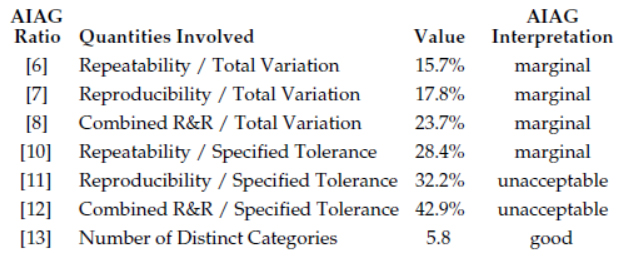Figure 3: Interpreting the results for the Gauge R&R Study for gasket thickness

Here we find that the measurement system used to measure the gaskets in figure 1 is simultaneously marginal, unacceptable, and good! Any questions?

While the ratios , , and  have the same numerators as ratios , , and  respectively, they have different denominators. In spite of these different denominators, the same guidelines are used for each ratio in the AIAG Gauge R&R Study. This is presumably one of the benefits of using guidelines that have absolutely no contact with reality. Since no justification for these guidelines exists, they can be adapted to fit any set of ratios that might be computed. After all, when attempting to interpret patent nonsense, it is always best to use arbitrary guidelines.

The inconsistency of the results in figure 3 is an inherent feature of the AIAG Gauge R&R Study. Once you ignore the Pythagorean theorem, mere guidelines are not going to remedy the sit­uation. After finding reasonable estimates for repeatability, reproducibility, combined R&R, product variation, and the total variation with formulas  through , the eight ratios  through  computed in the AIAG Gauge R&R Study are erroneous, fallacious, naive, incorrect, and, to put it quite simply, wrong.### But how do these guidelines match up with the four classes of process monitors?

In “The Intraclass Correlation Coefficient” (Quality Digest, Dec. 2, 2010) four classes of process monitors were defined. To see how the Gauge R&R Guidelines compare with the four classes of process monitors we need to begin by noting that the ratio in formula  is 100 times the following:Thus, if we plotted values for ratio  on one scale (in ascending order), and plotted values for the intraclass correlation on another scale (in descending order) we could connect equivalent points on the two scales to get figure 4.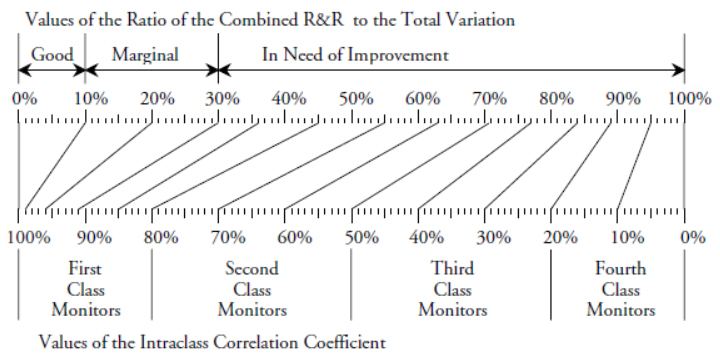Figure 4: The AIAG Guidelines and the four classes of process monitorsFigure 5: The four classes of process monitors defined by the intraclass correlation

The AIAG Gauge R&R Guidelines will pronounce a measurement system to be “good” only when it has an intraclass correlation in excess of 0.99; “marginal” measurement systems will have an intraclass correlation between 0.91 and 0.99; and everything else is “in need of improvement.” Contrast this to the results from the December 2010 column which are summarized in figure 5. There we see that even third class monitors can be used to track process improvements.

When we compare the results in figures 4 and 5 it becomes clear that the main purpose of the AIAG Gauge R&R Study is to condemn the measurement pro­cess. To achieve this end the summary ratios  through  are inflated so as to overstate the dam­age of measurement error while the guidelines used to interpret these inflated ratios are excessively conservative.

So what can you learn from the AIAG Gauge R&R Study? Virtually noth­ing that is true, correct, or useful! You have taken the time and gone to the trouble to collect good data, and then you have wasted the information contained in those data by performing a hopelessly flawed analysis.

### Summary

The Pythagorean theorem would need to be repealed before the summary ratios  through  of the AIAG Gauge R&R Study could even begin to make sense. Since that is unlikely to happen anytime soon, you need to stop using the erroneous ratios of the AIAG Gauge R&R Study.

The number of distinct categories value from ratio  does not represent anything that can be expressed in practical terms. So even though I may be the author of this ratio, it is useless in practice. I personally quit using it back in the 1980s. I suggest that you do the same, starting immediately.

The erroneous ratios , , and  overstate the impact of measurement error upon specifications. As shown in last month’s column, “More About the Precision to Tolerance Ratio,” the actual relationship between measurement error and specifications is much more complex than these ratios imply. Large values for ratios , or  cannot be used to judge the utility of the measurement process.

Honest ratios [Honest Ratio 6] through [Honest Ratio 9] replace the erroneous ratios  through . Honest ratio 9 is an estimate of the traditional, theoretically sound, intraclass correlation coefficient which correctly defines the relative utility of a measurement process for a given application.

Since many software packages currently give the nonsense AIAG ratios as part of their “measurement system analysis” you will need to identify these fallacious numbers on the output in order to avoid being misled by them.

This article and my earlier articles provide you with practical, theoretically sound, and easy-to-use alternatives to the nonsense ratios of the AIAG Gauge R&R Study. They allow you to learn and use the correct formulas for evaluating the measurement process. Further documentation and explanation can be found in my book EMP III: Evaluating the Measurement Process and Using Imperfect Data (SPC Press, 2006).

However, if you still want to obfuscate and confuse the issues, if you want to needlessly condemn measurement systems, and if you want to continue to beat vendors over the head, then by all means continue to use the AIAG Gauge R&R Study where all the results are untrue, inappropriate, and wrong.

### Discuss### Donald J. Wheeler

Dr. Wheeler is a fellow of both the American Statistical Association and the American Society for Quality who has taught more than 1,000 seminars in 17 countries on six continents. He welcomes your questions; you can contact him at djwheeler@spcpress.com.

### Gauge R&R

Suppliers will complain that their customers want the GR&R results using AIAG formulas.

Fortunately, it is easy to calculate the true results and provide them along side the AIAG ones.

Dr. Wheeler,  This article was both insightful and comprehensive. I never miss one of your articles in Quality Digest. Thank you for continuing to educate and lead the quality profession.

Sincerely,

Jonathan Boyer

### # of Distinct Categories

When you consider the range of values submitted to the Gage, I find that the number of distinct categories assists in explaining the cloud of uncertainty for a given value. Cloud = range / # of categories.

### AIAG MSA Studies

Good article!  I'd like to hear your take on a lesser known and practiced AIAG analysis, the (simple) Gage R.  In the Gage R study, 10 measurements of a single characteristic (one part!) are taken by a single operator using a single measurement system.  This would seem to eliminate part variation and (multiple) operator variation.  The result being a distribution of the 10 measurements unsullied by operator (1, 2, or 3) differences, gage  (micrometer?, calipers? CMM / CMM Program?) differences, or actual variation between parts (#1-#5).  (What is included is "Within Part Variation", for example the difference in the thickness of the gasket at different locations.  This variation can be minimized by requiring that the repeated measurement always be taken at a specific location.)

The Gage R analysis has been used extensively in auto-body panel production where it is important to reduce the spread of the 10 measurement distribution as part of the gage development long before quantities of production parts even exist.  Note that these are not "easy" parts.  They have few if any flat surfaces, are flexible far beyond their dimensional tolerances, and are likely to flex and/or move under measurement forces.  Sophisticated holding fixtures (and a CMM) or gages (with integrated data collection) are essential for their measurement.  Measurements are taken relative to coordinate systems structured around datums established from datum features designated on the parts.

The variation in the 10 measurements is sometimes severe.  Depending on where and in what direction the measurements vary some conclusions for possible improvements can usually be made.  Sometimes the part is moving on locator pins.  Sometimes it is flexing (differently) each time it is measured.  The insights gained by the analysis can even lead to tooling improvements that improve assembly fit and quality through the life of the program.

### Problems With the AIAG Gauge R&R Study

Thank you, Dr. Wheeler. Finally someone with the statistical credentials has said "the emperor has no clothes". Maybe now we can get AIAG to correct their error.

### Also the RPN

Dr. Wheeler also pointed out the problem with the Risk Priority Number from FMEA, as it is the product of three ordinal numbers. The newest AIAG/VDA FMEA manual no longer uses it and has replaced it with an Action Priority. It is a major improvement on the previous method.

This article also has valuable takeaways. We need to know what is and is not of immediate practical value. I think the gage standard deviation (appraiser and equipment variation combined) is the most important because we can use it to calculate the chance of passing bad product and scrapping good product. The ratios, as Dr. Wheeler points out, are not as informative.